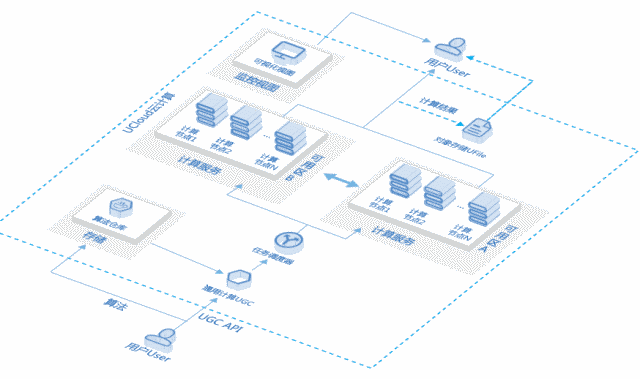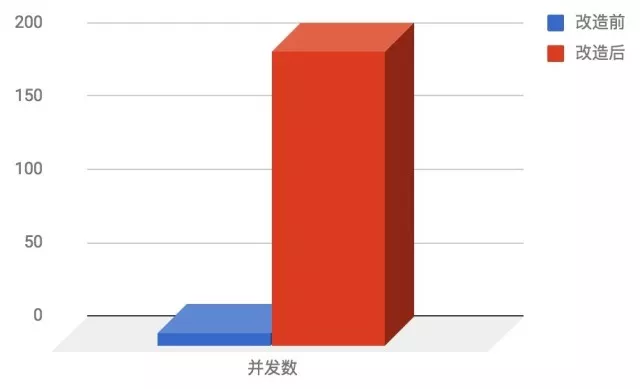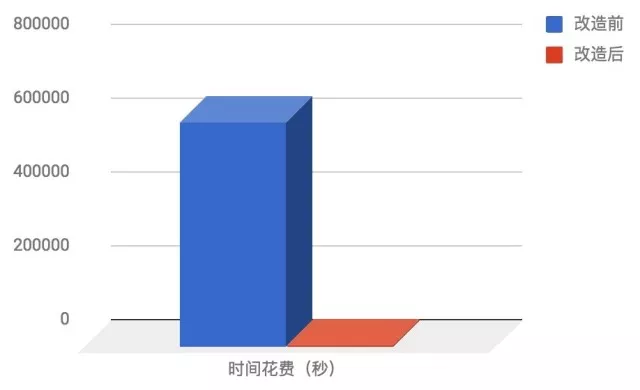# 一次 Serverless 架构改造实践：基因样本对比Serverless 是一种新兴的无服务器架构，使用它的时候开发者只需专注于代码，无需关心运维、资源交付或者部署。本文从代码的角度，通过改造一个 Python 程序来帮助读者从侧面理解 Serverless，让应用继承 Serverless 架构的优点。

## 现有资源：

1. 一个成熟的基因对比算法（Python 实现，运行一次的时间花费为 2 秒）
2. 2020 个基因样本文件（每个文件的大小为 2M，可以直接作为算法的输入）
3. 一台 8 核心云主机

### 基因检测服务

├── relation.py └── samples       ├── one.sample   └── two.sample relations.py 代码如下： import sys def relationship_algorithm(human_sample_one, human_sample_two):   # it's a secret   return result if __name__ == "__main__":   length = len(sys.argv)     # sys.argv is a list, the first element always be the script's name   if length != 3:       sys.stderr.write("Need two samples")     else:             # read the first sample       with open(sys.argv, "r") as sample_one:           sample_one_list = sample_one.readlines()        # read the second sample       with open(sys.argv, "r") as sample_two:           sample_two_list = sample_two.readlines()        # run the algorithm       print relationship_algirithm(sample_one_list,                 sample_two_list)

➜ python relation.py ./samples/one.sample ./samples/two.sample ➜ 0.054

## 我们接到了如下业务需求：

2000 * 20 = 40000（次）

40000（次） * 2（秒）= 80000 （秒）80000（秒）/ 60.0 / 24 ≈ 56（天）

56 / 8 ≈ 7（天）

## 介绍一种 Serverless 产品：UGC

UGC: UCloud General Compute（通用计算）1. 算法镜像的名字和一些验证信息通过 querystring 的形式 （例如： http://api.ugc.service.ucloud.cn?ImageName=relation&AccessToken=！Q@W#E）

2. 算法镜像所需的数据通过 HTTP body 的形式

## 针对此 Serverless 架构的改造

1. 改造算法中元数据从「文件输入」改为「标准输入」，输出改为「标准输出」。
2. 开发客户端构造 HTTP 请求，并提高并发。

### 01 改造算法输入输出

① 改造输入为 stdin

cat ./samples/one.sample ./samples/two.sample    | python relation.py

import sysmystdin = sys.stdin.read() # 这里的 mystdin 包含 ./samples/one.sample ./samples/two.sample 的全部内容，无分隔，实际使用可以自己设定分隔符来拆分。

② 将算法的输出数据写入 stdout

# 把标准输入拆分回两个 samplesample_one, sample_two = separate(mystdin)# 改造算法的输出为 stdoutdef relationship_algorithm(sample_one,    sample_two)   # 改造前   return result   # 改造后   sys.stdout.write(result)

### 02 客户端与开发

imageName =  "cn-bj2.ugchub.service.ucloud.cn/testbucket{1}/relationship:0.1" token = tokenManager.getToken()  # SDK 有现成的 # summitTask 构造 HTTP 请求并将镜像的 STDOUT 打成 tar 包返回 response = submitTask(imageName, token, data)

import tarfile import io def untar(data):   tar = tarfile.open(fileobj=io.BytesIO(data))     for member in tar.getmembers():       f = tar.extractfile(member)         with open('result.txt','a')  as resultf:           strs = f.read()           resultf.write(strs)

sample_2000_list,  sample_20_list = get_sample_list()

import itertoolsall = list(itertools.product(sample_2000_list, sample_20_list)) assert len(all) == 40000

def worker(two_file_tuple):   sample_one_dir, sample_two_dir = two_file_tuple    with open(sample_one_dir) as onef:       one_data = onef.read()     with open(samle_two_dir) as twof:       two_data = twof.read()   data = one_data + two_data   response = summitTask(imageName, token, data)   untar(response)

import gevent.pool import gevent.monkeygevent.monkey.patch_all() # 猴子补丁pool = gevent.pool.Pool(200)pool.map(worker, all)40000 * 2 = 80000（秒）
80000 / 200 = 400（秒）1. 免运维 – 因为你没有服务器了…

2. 高可用 – Serverless 服务一般依托云计算的强大基础设施，任何模块都不会只有单点，都尽可能做到跨可用区，或者跨交换机容灾，而且本次使用的服务有一个有趣的机制：同一个任务，你提交一次，会被多个节点执行，如果一个计算节点挂了，其他节点还可以正常返回，哪个先执行完，先返回哪个。

3. 按需付费 – 文中说每个算法执行一次花费单核心 CPU 时间 2 秒，我们直接算一下花费：

2000 * 20 * 2 = 80000（秒）
80000 / 60 / 24.0 = 55.5556（小时）
55.5556（小时） * 0.09（元） * 1（核心） ~
= 5（元）

4. 发布简单 – 因为使用 Docker 作为载体，所以它是语言无关的，而且发布也很快，代码写好直接上传镜像就好了，至于灰度，客户端 imageName 指定不同版本即可区分不同代码了。

https://mp.weixin.qq.com/s/Hw3pb8y7Q-g0QsevvG7sLQ